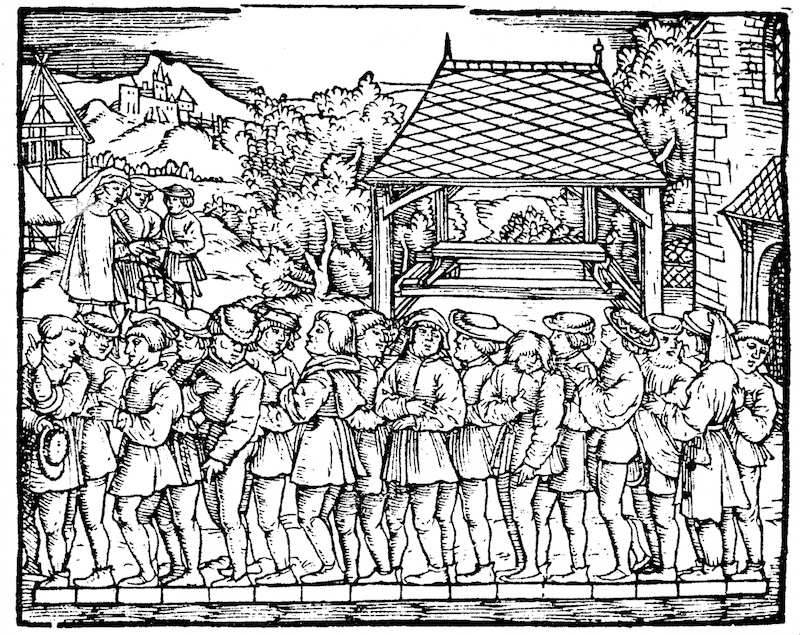# 2.5. Markdown Cells¶

The d2lbook provide additional features beyond the normal markdown supports in Jupyter.

You can use a toc code block to specify the table of contents. Here :maxdepth: 2 means display two levels of files, and :numbered: means adding number to each section (default is not enabled). Also note that you don’t need to specify the file extension.

toc
:maxdepth: 2
:numbered:

guide/index



## 2.5.2. Images¶

We can put the image caption in []. In addition, we can use :width: followed by its value in an inline block to specify the image width, similarly use :height:for height.

![Estimating the length of a foot](../img/koebel.jpg)
:width:400pxFig. 2.5.1 Estimating the length of a foot

### 2.5.2.1. SVG Images¶

We recommend you to use SVG images as much as you can. It is sharp and its size is small. But since Latex doesn’t support SVG images, if you want to build a PDF output, you need to install rsvg-convert. On Macos, you can simply brew install librsvg or sudo apt-get install librsvg2-bin for Ubuntu.Fig. 2.5.2 A LSTM cell in SVG

## 2.5.3. Tables¶

You can insert table caption before the table by starting it with a :. Note that you need to leave an empty line between the caption and the table itself.

: The number is computed by $z_{ij} = \sum_{k}x_{ik}y_{kj}$.

| Year | Number | Comment |
| ---  | --- | --- |
| 2018 | 100 | Good year |
| 2019 | 200 | Even better, add something to make this column wider |

Table 2.5.1 The number is computed by $$z_{ij} = \sum_{k}x_{ik}y_{kj}$$.

Year

Number

Comment

2018

100

Good year

2019

200

Even better, add something to make this column wider

If the Table caption number doesn’t show properly, you may need to update pandoc to the latest version.

## 2.5.4. Cross References¶

We often want to reference sections, figures, tables and equations in a book.

### 2.5.4.1. Referencing Sections¶

We can put a label immediately after the section title to allow this section to be referenced by its label. The label format is :label: followed by its label name in an inline code block.

### Referencing Sections
:label:my_sec3


Then we can reference this section through :ref: followed by label name in an inline code block

:ref:my_sec3 demonstrates how to reference a section.


Referencing Sections demonstrates how to reference a section.

Note that it displays the referenced section title with a clickable link. We can also use a numbered version by changing :num: to :numref:, e.g. :numref:my_sec3.

If the label is incorrect, say we put my_sec2 here, the build log will contains a warning such as

WARNING: undefined label: my_sec2


You can turn it into error by setting warning_is_error = True in config.ini.

Besides, we can cross reference label from other files as well, e.g. Section 2.6. This applies to figures, tables and equations as well.

### 2.5.4.2. Referencing Images¶

Similarly we can label an image and reference it later.

![A nice image with a cat and a dog.](../img/catdog.jpg)
:width:300px
:label:img_catdog

As can be seen from :numref:img_catdog,Fig. 2.5.3 A nice image with a cat and a dog.

As can be seen from Fig. 2.5.3, there is a cat and a dog.

### 2.5.4.3. Referencing Tables¶

:This a is very long table caption. It will breaks into several lines. And
contains a math equation as well. $z_{ij} = \sum_{k}x_{ik}y_{kj}$.

| Year | Number | Comment |
| ---  | --- | --- |
| 2018 | 100 | Good year |
:label:table

Refer to :numref:table

Table 2.5.2 This a is very long table caption. It will breaks into several lines. And contains a math equation as well. $$z_{ij} = \sum_{k}x_{ik}y_{kj}$$.

Year

Number

Comment

2018

100

Good year

Refer to Table 2.5.2

### 2.5.4.4. Referencing Equations¶

The difference here is that we need to use eqlabel instead of label. For example

$$\hat{\mathbf{y}}=\mathbf X \mathbf{w}+b$$
:eqlabel:linear

In :eqref:linear, we define the linear model.

(2.5.1)$\hat{\mathbf{y}}=\mathbf X \mathbf{w}+b$

In (2.5.1), we define the linear model.